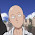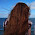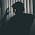## Monday, November 28, 2011

### No. 24 - Intersection of Sorted Arrays

Problem: Please implement a function which gets the intersection of two sorted arrays. Assuming numbers in each array are unique.

For example, if the two sorted arrays as input are {1, 4, 7, 10, 13} and {1, 3, 5, 7, 9}, it returns an intersection array with numbers {1, 7}.

Analysis: An intuitive solution for this problem is to check whether every number in the first array (denoted as array1) is in the second array (denoted as array2). If the length of array1 is m, and the length of array2 is n, its overall time complexity is O(m*n) based on linear search. We have two better solutions.

Solution 1: With O(m+n) Time

It is noticeable that the two input arrays are sorted. Supposing a number number1 in array1 equals to a number number2 in array2, the numbers after number1 in array1 should be greater than the numbers before number2 in array2. Therefore, it is not necessary to compare the numbers after number1 in array1 with numbers before number2 in array2. It improves efficiency since many comparisons are eliminated.

The sample code for this solution is shown below:

void GetIntersection_solution1(const vector<int>& array1,
const vector<int>& array2,
vector<int>& intersection)
{
vector<int>::const_iterator iter1 = array1.begin();
vector<int>::const_iterator iter2 = array2.begin();

intersection.clear();

while(iter1 != array1.end() && iter2 != array2.end())
{
if(*iter1 == *iter2)
{
intersection.push_back(*iter1);
++ iter1;
++ iter2;
}
else if(*iter1 < *iter2)
++ iter1;
else
++ iter2;
}
}

Since it only requires to scan two arrays once, its time complexity is O(m+n).

Solution 2: With O(nlogm) Time

As we know, a binary search algorithm requires O(logm) time to find a number in an array with length m. Therefore, if we search each number of an array with length n from an array with length m, its overall time complexity is O(nlogm). If m is much greater than n, O(nlogm) is actually less than O(m+n). Therefore, we can implement a new and better solution based on binary search in such a situation.

For instance, the following same code is suitable when array1 is much longer than array2.

/* === Supposing array1 is much longer than array2 === */
void GetIntersection_solution2(const vector<int>& array1,
const vector<int>& array2,
vector<int>& intersection)
{
intersection.clear();

vector<int>::const_iterator iter1 = array1.begin();
while(iter1 != array1.end())
{
if(binary_search(array2.begin(), array2.end(), *iter1))
intersection.push_back(*iter1);
}
}

The discussion about this problem is included in my book <Coding Interviews: Questions, Analysis & Solutions>, with some revisions. You may find the details of this book on Amazon.com, or Apress.

The author Harry He owns all the rights of this post. If you are going to use part of or the whole of this ariticle in your blog or webpages,  please add a reference to http://codercareer.blogspot.com/. If you are going to use it in your books, please contact me (zhedahht@gmail.com) . Thanks.

1.For solution 2, inserting array2 in a tree is O(mlogm). So the overall complexity will be O(nlom + mlogm) = O((n+m)logm), still greater than O(n+m). The latter is the best possible solution.

1.Hi Harry,

Your writing shines! There is no room for gibberish here clearly you have No. 24 - Intersection of Sorted Arrays with the next greatest. Keep writing!

Currently AWS and Amazon.com consumer accounts are bound together if you use your one and only email account to set up both. It is a complete breech of privacy to have to surrender my consumer Amazon.com account to move my AWS account to a business account. These should be able to be separated without taking down the production site AWS Training USA . The only option available now is manual migration which is silly considering the sophistication of AWS.

I read multiple articles and watched many videos about how to use this tool - and was still confused! Your instructions were easy to understand and made the process simple.

Cheers,
Kevin

2.for nonsorted arrays, it'd be beneficial to use specialized numerical sorts (flashsort is incredibly fast and efficient) and then do an element-wise comparison of the two arrays. if arrays are integer-domain, using a bitset then using AND on corresponding bits would be very fast also

3.The second solution works nicely even if the shorter array (here length n) is unsorted. If however, its the longer array that's unsorted, its better to sort that first and then proceed as before. The final complexity is then O(m.log(m) + n.log(m)) = O(log(m).(m+n))

4.Use hash table to store the first set of array elements, then iterate through the second array find(x) in the hash table created for the first array. It should also be O(m + n)

5.For solution 2, is it assuming array2 is much longer than array1? It looks like we're doing binary search on array2, while traversing array1

6.For the case when m is smaller than n, you can actually reduce the runtime cost to O(m * log(n/m)). For working code with extensive explanation, see this implementation.

7.Using Hashtable is also one solution that takes O(n+m) time but O(n+m) space as well.

8.in solution 2 you will need mlogm time to construct the tree hence total complexity is (m+n)logm which is greater then n+m

9.Hi There,

Brilliant article, glad I slogged through the No. 24 - Intersection of Sorted Arrays it seems that a whole lot of the details really come back to from my past project.

It came to my attention that several links to the Qwiklab-hosted labs are no longer working.
e.g. In Data Scientist track's AWS Cloud Computing module , "AWS Analytiacs" page, the links to ES and EMR labs lead to Qwiklab page with error message basically stating it is "currently unavailable". (I was logged into Qwiklab already at the point).

It was cool to see your article pop up in my google search for the process yesterday. Great Guide.
Keep up the good work!

Ciao,
Preethi.

10.Howdy Mate,

Your writing shines like gold! There is no room for gibberish here clearly. You nailed it in No. 24 - Intersection of Sorted Arrays !

I need something where I can run iis, sql server express and an application server to deploy a program. I thought I could use lightsail but I don't see an option to install the application server. Should I be using something else? AWS Training USA

Anyways great write up, your efforts are much appreciated.

Best Regards,